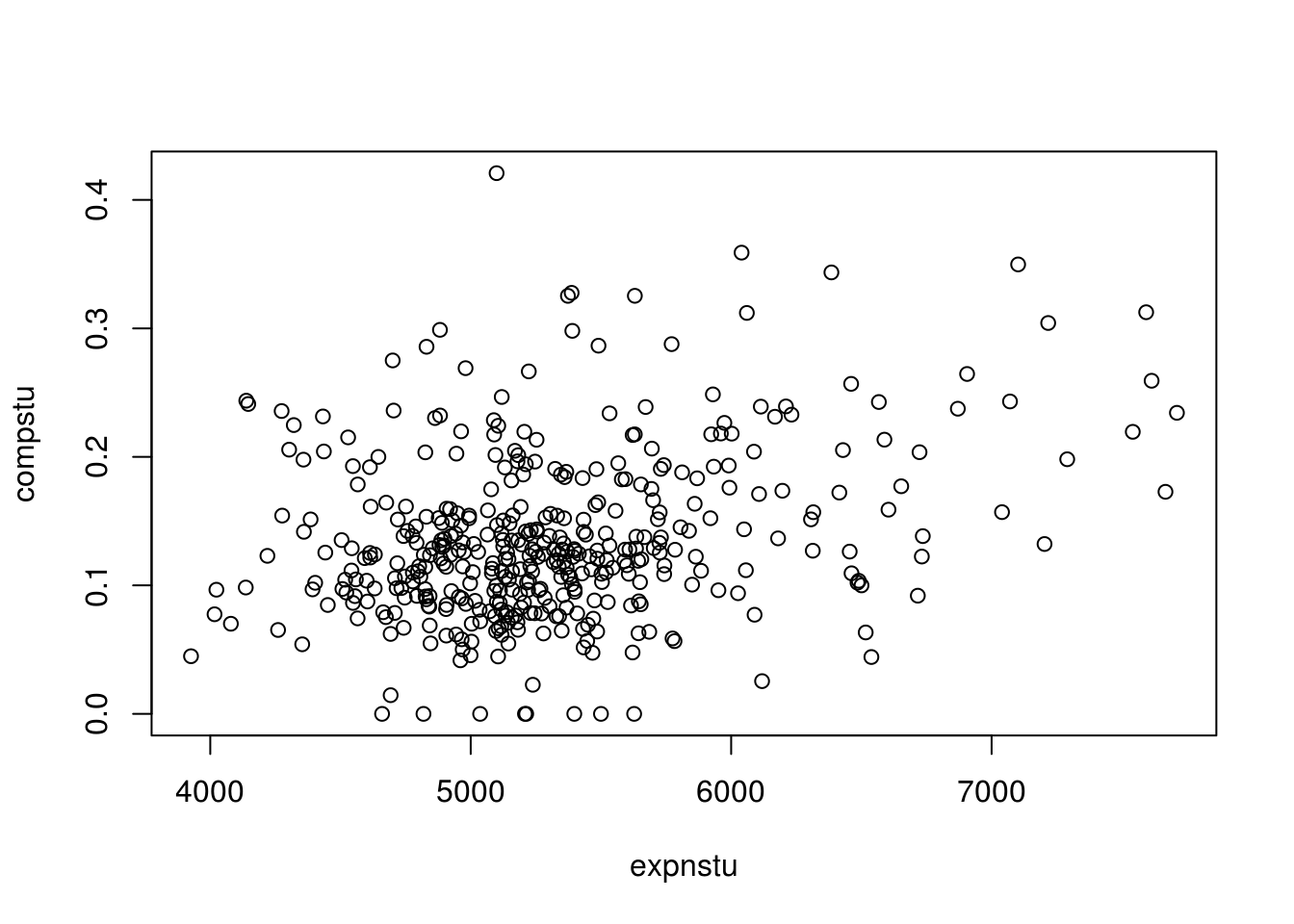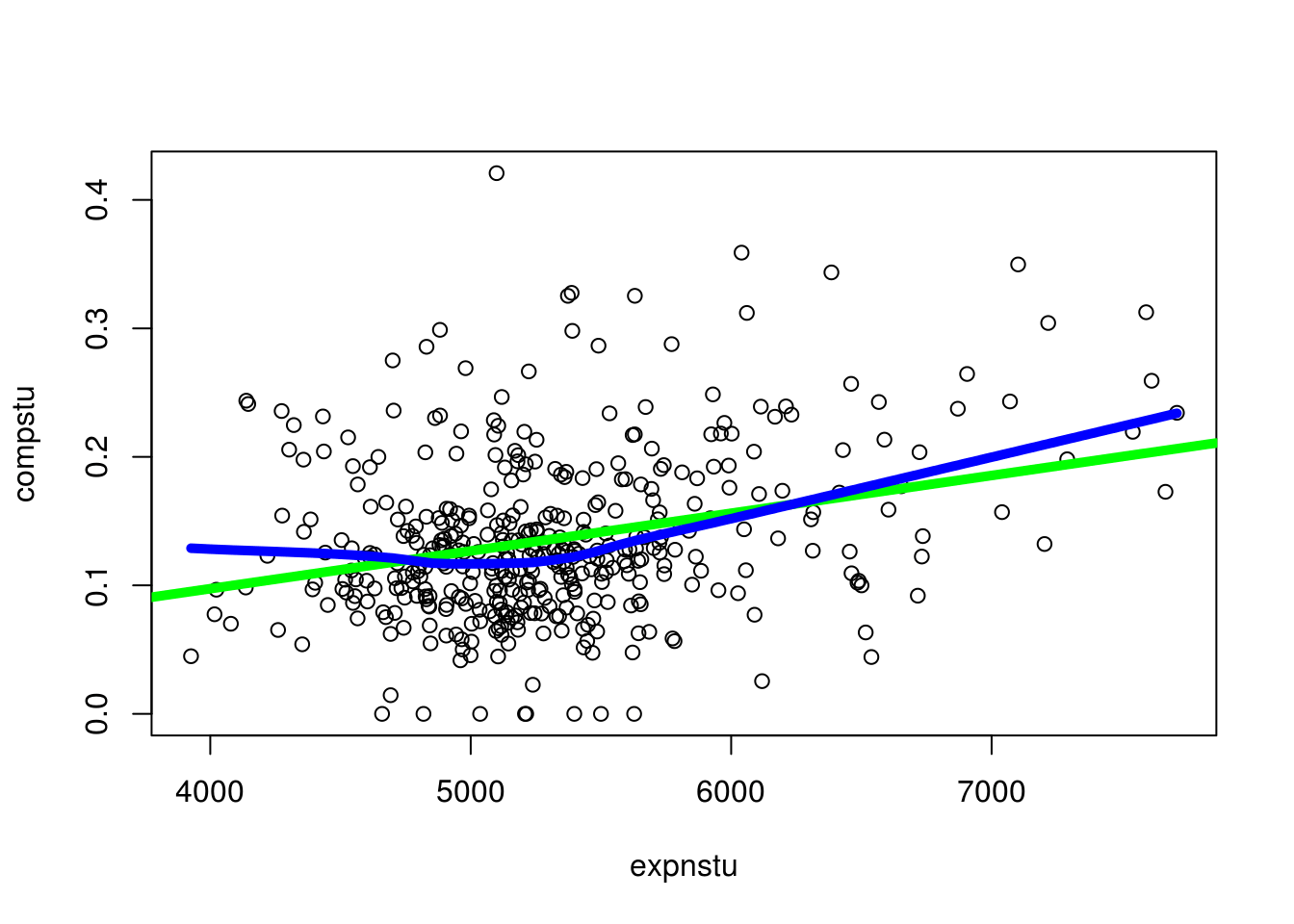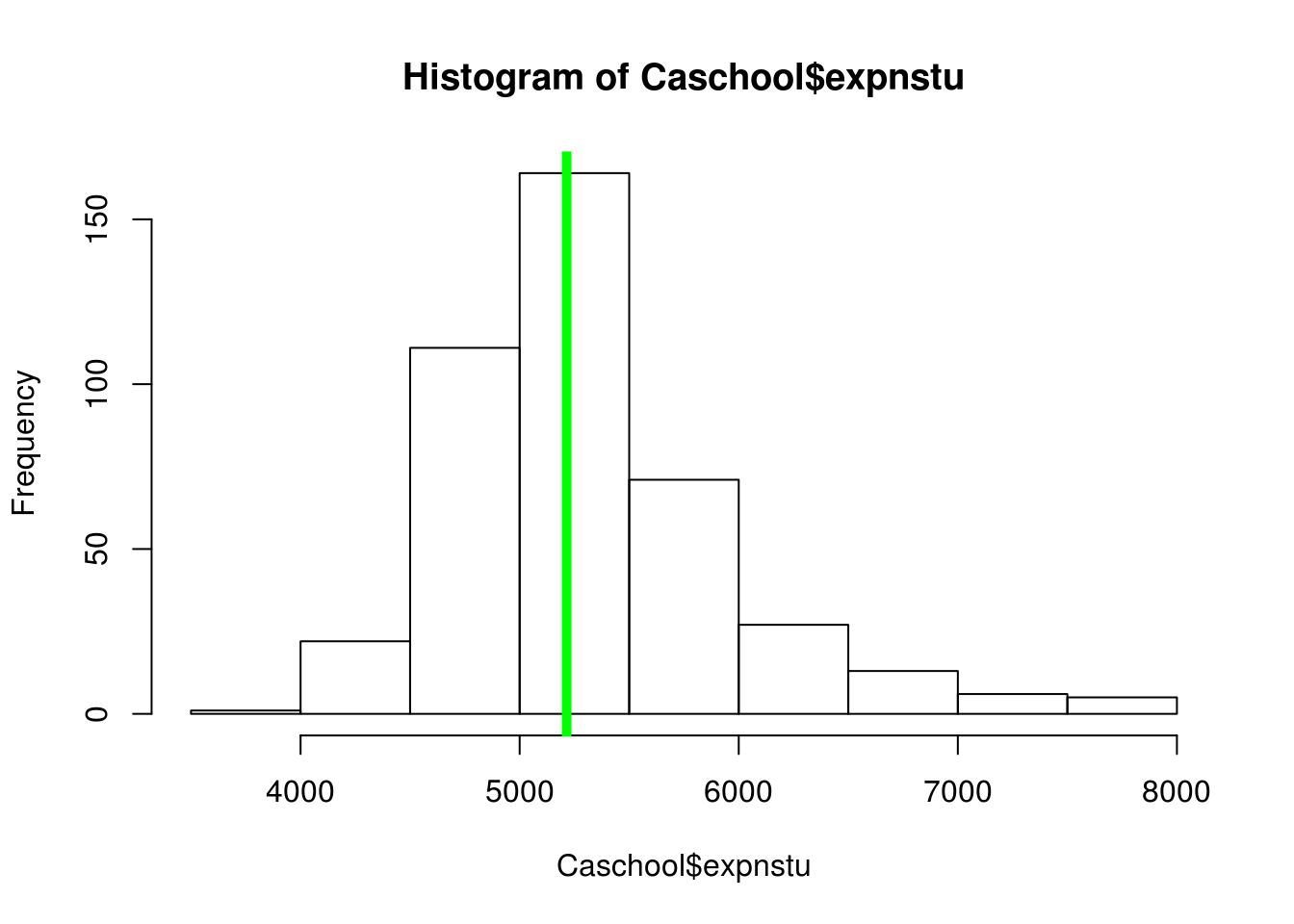# Making Graphics in R

Data visualization is a critical component of communicate results with an audience. Fortunately, R provides many different ways to present numerical data it a clear way visually. This post will look specifically at making data visualizations with the base r package “graphics”.

Generally, functions available in the “graphics” package can be either high-level functions or low-level functions. High-level functions actually make the plot. Examples of high-level functions includes are the “hist” (histogram), “boxplot” (boxplot), and “barplot” (bar plot).

Low-level functions are used to add additional information to a plot. Some commonly used low-level functions includes “legend” (add legend) and “text” (add text). When coding we allows call high-level functions before low-level functions as the other way is not accepted by R.

We are going to begin with a simple graph. We are going to use the“Caschool” dataset from the “Ecdat” package. For now, we are going to plot the average expenditures per student by the average number of computers per student. Keep in mind that we are only plotting the data so we are only using a high-level function (plot). Below is the code

``library(Ecdat)``
``````data("Caschool")
plot(compstu~expnstu, data=Caschool)``````The plot is not “beautiful” but it is a start in plotting data. Next, we are going to add a low-level function to our code. In particular, we will add a regression line to try and see the diretion of the relationship between the two variables via a straight line. In addition, we will use the “loess.smooth” function. This function will allow us to see the general shape of the data. The regression line is green and the loess smooth line is blue. The coding is mostly familiy but the “lwd” argument allows us to make the line thicker.

``````plot(compstu~expnstu, data=Caschool)
abline(lm(compstu~expnstu, data=Caschool), col="green", lwd=5)
lines(loess.smooth(Caschool\$expnstu, Caschool\$compstu), col="blue", lwd=5)``````Boxplots allow you to show data that has been subsetted in some way. This allows for the comparisions of groups. In addition, one or more boxplots can be used to identify outliers.

In the plot below, the student-to-teacher ratio of k-6 and k-8 grades are displayed.

``boxplot(str~grspan, data=Caschool)``As you look at the data you can see there is very little difference. However, one major differnce is that the K-8 group has much more extreme values than K-6.

Histograms are an excellent way to display information about one continuous variable. In the plot below, we can see the spread of the expenditure per student.

``hist(Caschool\$expnstu)``We will now add median to the plot by calling the low-level function “abline”. Below is the code.

``````hist(Caschool\$expnstu)
abline(v=median(Caschool\$expnstu), col="green", lwd=5)``````Conclusion

In this post, we learned some of the basic structures of creating plots using the “graphics” package. All plots in include both low and high-level functions that work together to draw and provide additional information for communicating data in a visual manner